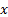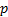/

### The geometric distribution

The geometric distribution describes the probability ofsuccesses in a sequence of independent experiments each with likelihood of success ofthat arise before there is 1 failure. It is a special case of the negative binomial distribution.

Note: different texts adopt slightly different definitions, e.g. it may be the total number of trials (i.e. 1 more than the above) in which case it may be called the shifted geometric distribution and/ormay denote the probability of failure rather than the probability of success.